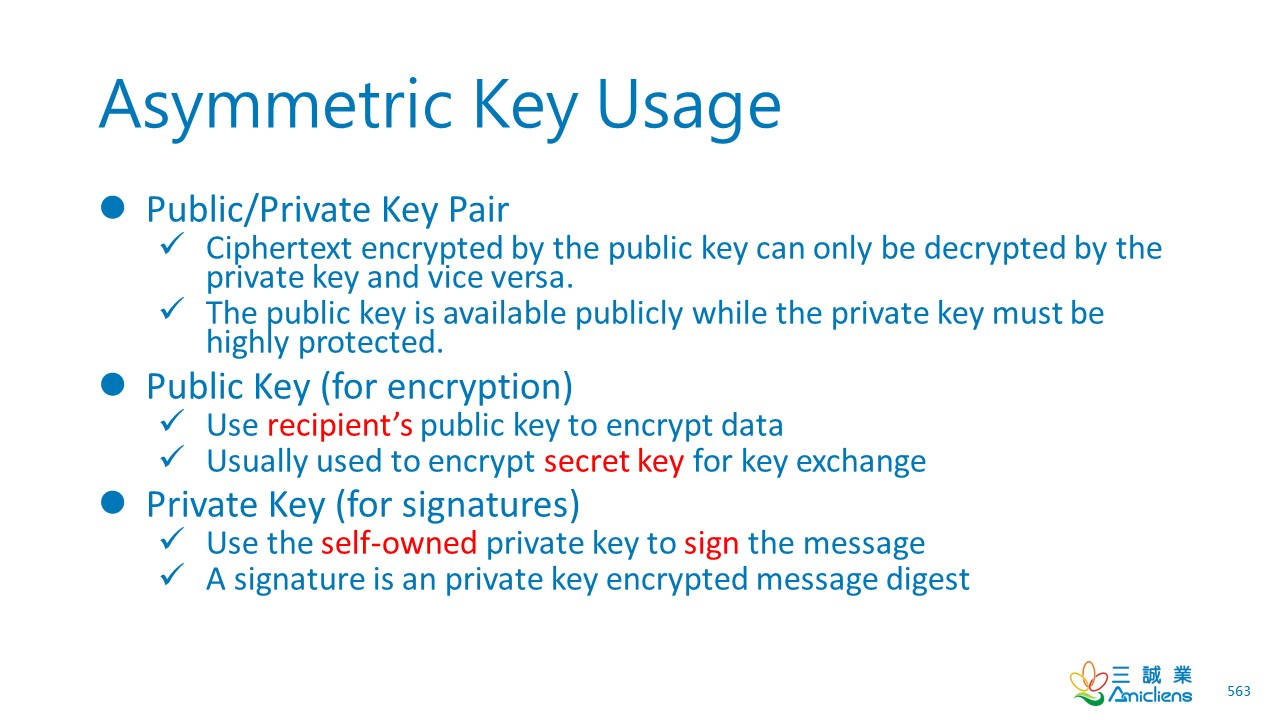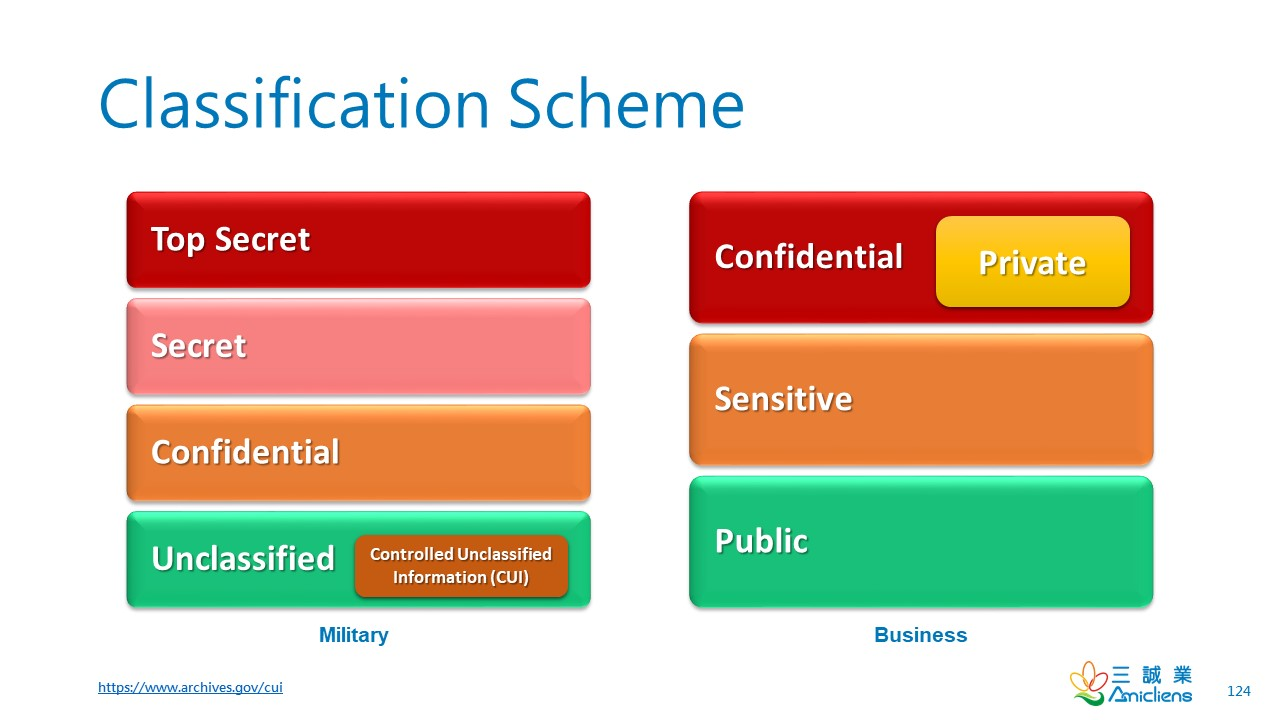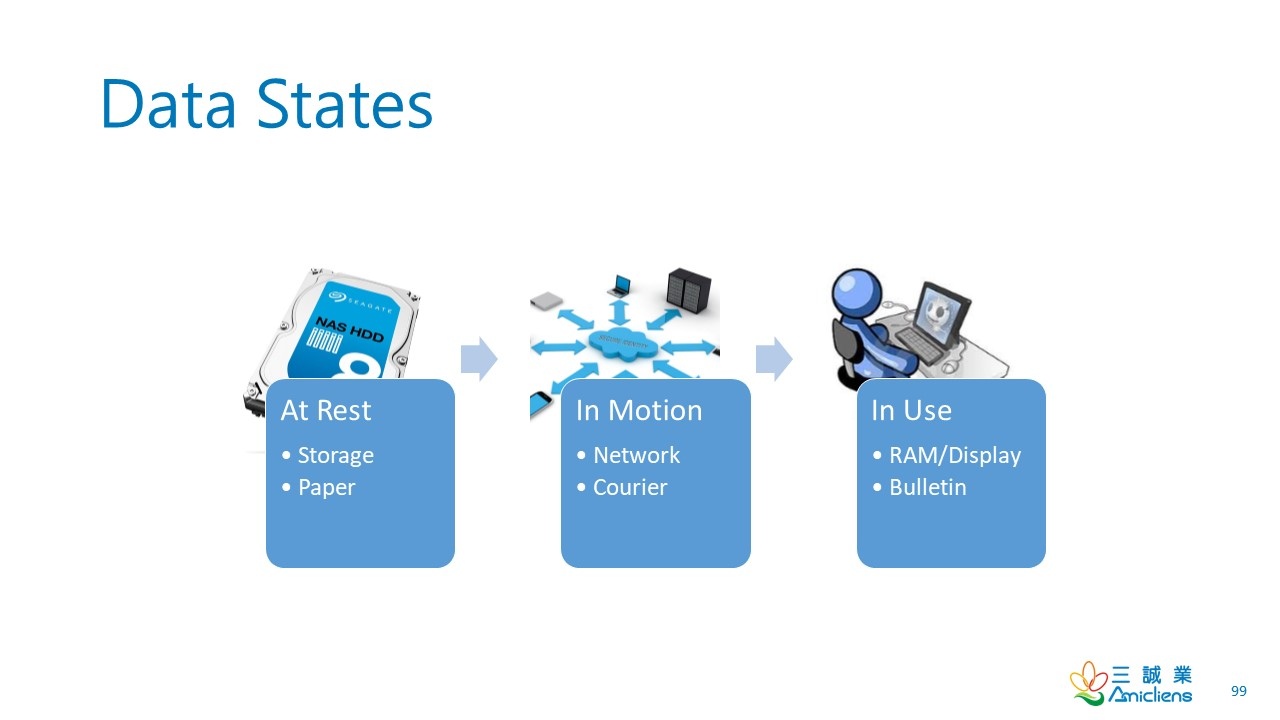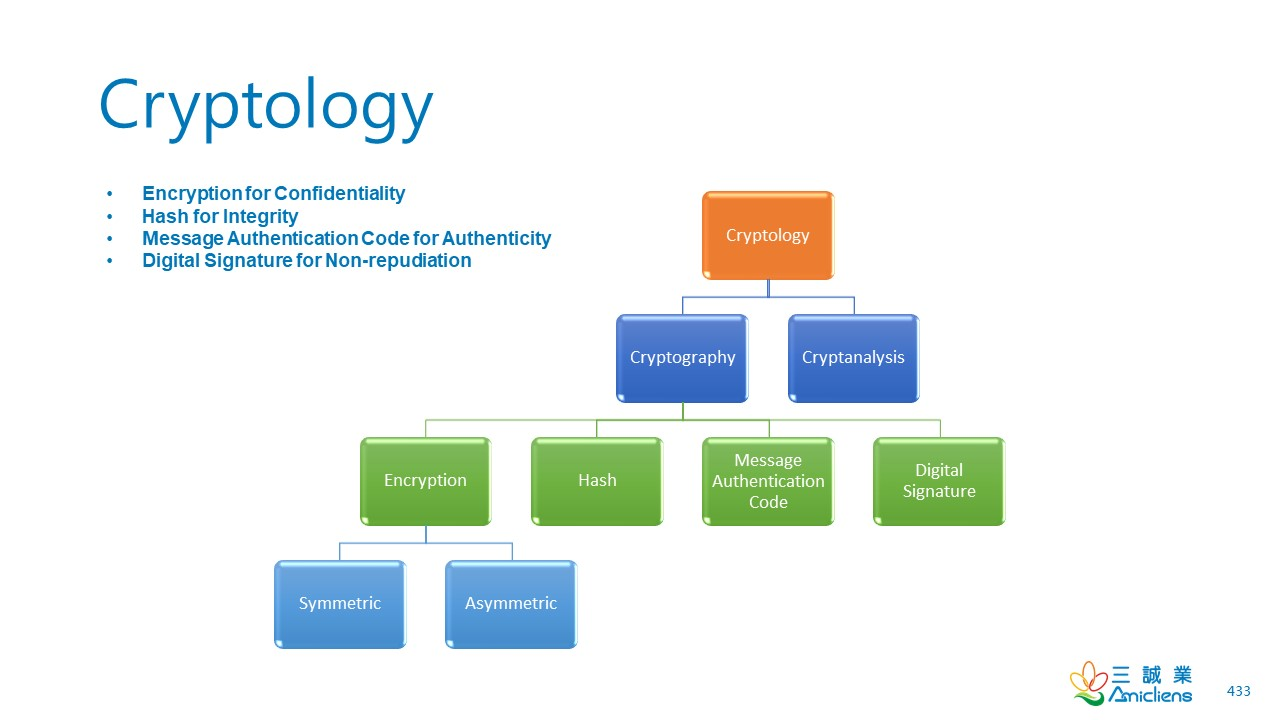# CISSP PRACTICE QUESTIONS – 20190905Alice is tasked to evaluate and implement a cryptographic solution to protect the company’s classified data at rest, in motion, and in use accross the data life cycle. She decides to use a hybrid strategy, that is, the synergy of symmetric and asymmetric cryptography.  The asymmetric cryptography is used for symmetric key exchange and digital signature, while the data is protected by symmetric cryptography. Which of the following is the most unlikely to achieve in terms of her strategy?
A. Confidentiality
B. Integrity
C. Availability
D. Non-repudiation

Kindly be reminded that the suggested answer is for your reference only. It doesn’t matter whether you have the right or wrong answer. What really matters is your reasoning process and justifications.

My suggested answer is C. Availability.## Key Size and Cryptographic Strength

3072-bit RSA keys are equivalent in strength to 128-bit symmetric keys and 256-bit  ECC keys (elliptic curve cryptography).

• Symmetric > Asymmetric (strength )
• ECC > RSA (strength)
• ECC = Symmetric * 2 (key size)

As of 2003 RSA Security claims that 1024-bit RSA keys are equivalent in strength to 80-bit symmetric keys, 2048-bit RSA keys to 112-bit symmetric keys and 3072-bit RSA keys to 128-bit symmetric keys.

NIST guidelines state that ECC keys should be twice the length of equivalent strength symmetric key algorithms. So, for example, a 224-bit ECC key would have roughly the same strength as a 112-bit symmetric key. (Wikipedia: Key Size)

It is the most serious problem that Alice sent to bob a document encrypted by her private key. As Alice’s public key is publicly available, everyone can decrypt the encrypted document sent to Bob. It causes a data breach.This post is copied from the second question in CISSP PRACTICE QUESTIONS – 20190904, which has two questions, to serve as the QOTD of 20190905 on 20200816.